# How Apriori Algorithm works?

### Steps for Apriori Algorithm

Below are the steps for the apriori algorithm:

Step-1: Determine the support of itemsets in the transactional database, and select the minimum support and confidence.

Step-2: Take all supports in the transaction with higher support value than the minimum or selected support value.

Step-3: Find all the rules of these subsets that have higher confidence value than the threshold or minimum confidence.

Step-4: Sort the rules as the decreasing order of lift.

### Apriori Algorithm Working

We will understand the apriori algorithm using an example and mathematical calculation:

Example: Suppose we have the following dataset that has various transactions, and from this dataset, we need to find the frequent itemsets and generate the association rules using the Apriori algorithm: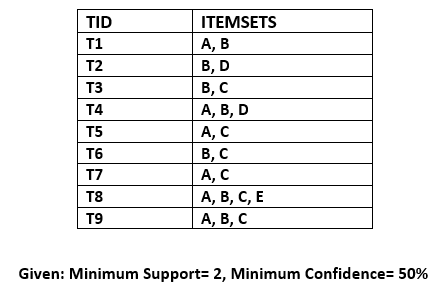### Step-1: Calculating C1 and L1:

• In the first step, we will create a table that contains support count (The frequency of each itemset individually in the dataset) of each itemset in the given dataset. This table is called the Candidate set or C1.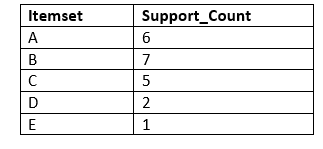• Now, we will take out all the itemsets that have the greater support count that the Minimum Support (2). It will give us the table for the frequent itemset L1.
Since all the itemsets have greater or equal support count than the minimum support, except the E, so E itemset will be removed.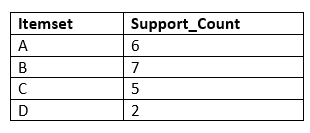### Step-2: Candidate Generation C2, and L2:

• In this step, we will generate C2 with the help of L1. In C2, we will create the pair of the itemsets of L1 in the form of subsets.
• After creating the subsets, we will again find the support count from the main transaction table of datasets, i.e., how many times these pairs have occurred together in the given dataset. So, we will get the below table for C2: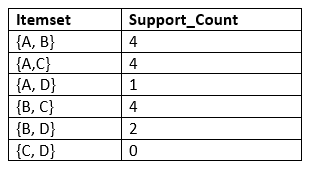• Again, we need to compare the C2 Support count with the minimum support count, and after comparing, the itemset with less support count will be eliminated from the table C2. It will give us the below table for L2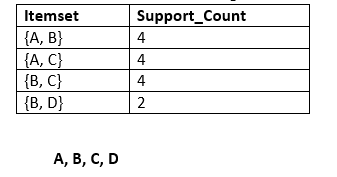### Step-3: Candidate generation C3, and L3:

• For C3, we will repeat the same two processes, but now we will form the C3 table with subsets of three itemsets together, and will calculate the support count from the dataset. It will give the below table: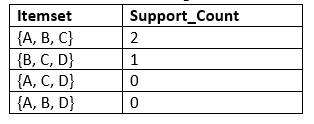• Now we will create the L3 table. As we can see from the above C3 table, there is only one combination of itemset that has support count equal to the minimum support count. So, the L3 will have only one combination, i.e., {A, B, C}.

### Step-4: Finding the association rules for the subsets:

To generate the association rules, first, we will create a new table with the possible rules from the occurred combination {A, B.C}. For all the rules, we will calculate the Confidence using formula sup( A ^B)/A. After calculating the confidence value for all rules, we will exclude the rules that have less confidence than the minimum threshold(50%).

Consider the below table:

Rules Support Confidence
A ^B → C 2 Sup{(A ^B) ^C}/sup(A ^B)= 2/4=0.5=50%
B^C → A 2 Sup{(B^C) ^A}/sup(B ^C)= 2/4=0.5=50%
A^C → B 2 Sup{(A ^C) ^B}/sup(A ^C)= 2/4=0.5=50%
C→ A ^B 2 Sup{(C^( A ^B)}/sup©= 2/5=0.4=40%
A→ B^C 2 Sup{(A^( B ^C)}/sup(A)= 2/6=0.33=33.33%
B→ B^C 2 Sup{(B^( B ^C)}/sup(B)= 2/7=0.28=28%

As the given threshold or minimum confidence is 50%, so the first three rules A ^B → C, B^C → A, and A^C → B can be considered as the strong association rules for the given problem.

Apriori is an algorithm which determines frequent item sets in a given datum.

Lets say you have gone to supermarket and buy some stuff. The following would be in the screen of the cashier

User : X1

ID : Item
1 : Cheese
2. : Biscuits
3. : Pop tarts
4. : Ramen Noodles

This data is stored into their database as one transaction. ie. the User X1 has bought these many items in one go. Like this the database separately stores a set of items bought by customers { X1 … Xn). For example .

## X1: {1,2,3,4} X2: {1,2} X3: {2,4} X4: {3,4} X5: {1,2,4}

Let say frequency threshold = 2 . ie. if a given data item has occurred 2 or more number of times, its said to be frequent.

Step 1:
Find Frequent Singles in item_sets
{1} : 3
{2} : 4
{3} : 2
{4} : 4

Step 2:
Find Frequent Doubles in item_sets

{1,2}: 3
{1,3}: 1
{1:4}: 2
{2,3}: 1
{2,4}: 3
{3,4}: 2

We can leave sets {1,3} and {2,3} since they are below the frequency threshold.
So any larger set containing {1,3} and {2,3} will not be considered.

Step 3:
Find Frequent Triples in item_sets
{1,2,4} : 2

you stop here . Since you cannot go any further. Thus the frequent triplets are {1,2,4}. Since all other triplets are excluded due to the fact that {1,3} and {2,3} cannot be considered.

Apriori algorithm is pretty old algorithm to mine information with respect to frequent data occurrences in a given database or stream data. That said, its the easiest algorithm to implement.